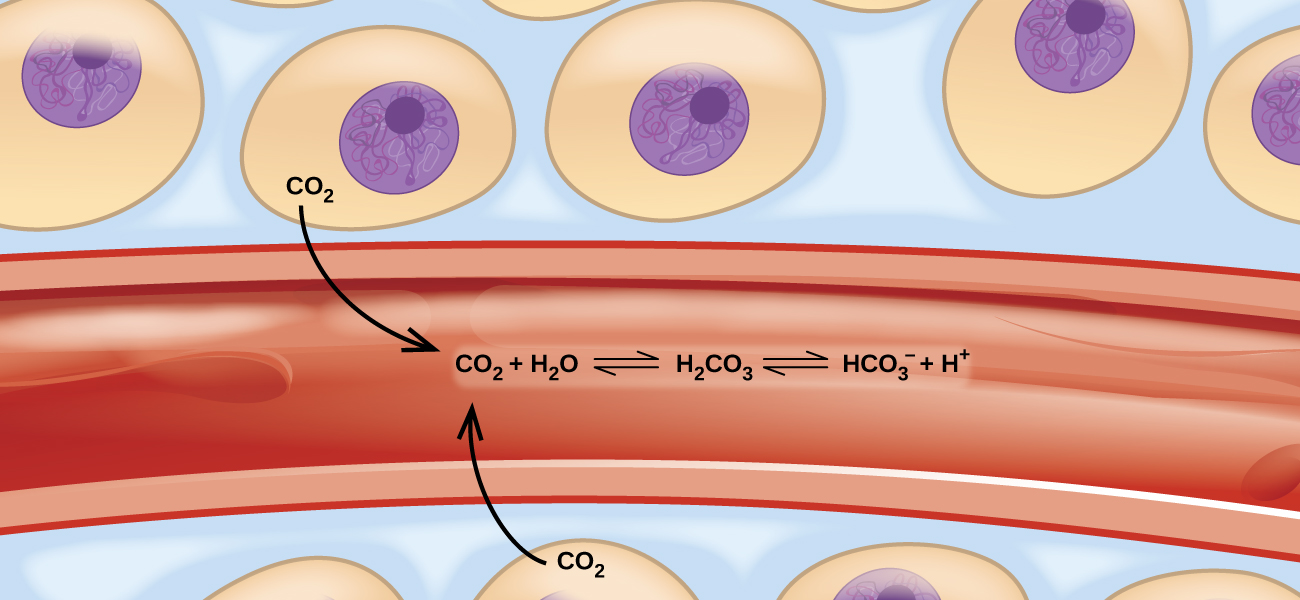# 6.1 Introduction

Learning Objectives

• Chemical Equilibria
• Equilibrium Constants
• Shifting Equilibria: Le Châtelier’s Principle
• Equilibrium CalculationsFigure 6.1.1 – Transport of carbon dioxide in the body involves several reversible chemical reactions, including hydrolysis and acid ionization (among others).

Imagine a beach populated with sunbathers and swimmers. As those basking in the sun get too hot, they enter the surf to swim and cool off. As the swimmers tire, they return to the beach to rest. If the rate at which sunbathers enter the surf were to equal the rate at which swimmers return to the sand, then the numbers (though not the identities) of sunbathers and swimmers would remain constant. This scenario illustrates a dynamic phenomenon known as equilibrium, in which opposing processes occur at equal rates. Chemical and physical processes are subject to this phenomenon; these processes are at equilibrium when the forward and reverse reaction rates are equal. Equilibrium systems are pervasive in nature; the various reactions involving carbon dioxide dissolved in blood are examples (see (Figure 6.1.1)). This chapter provides a thorough introduction to the essential aspects of chemical equilibria.